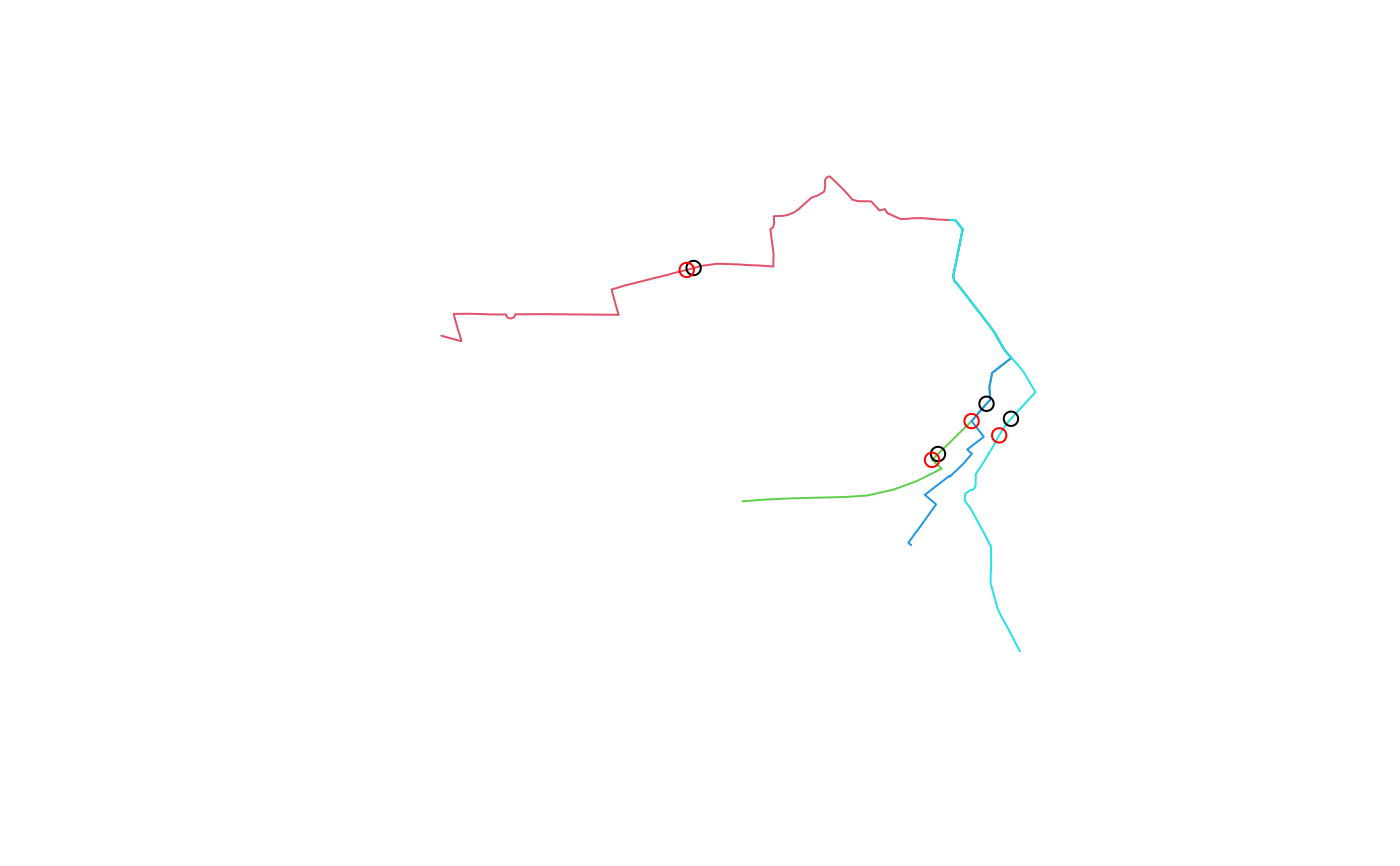Find the mid-point of lines

## Usage

``line_midpoint(l, tolerance = NULL)``

## Arguments

l

A spatial lines object

tolerance

The tolerance used to break lines at verteces. See `lwgeom::st_linesubstring()`.

Other lines: `angle_diff()`, `geo_toptail()`, `is_linepoint()`, `line2df()`, `line2points()`, `line_bearing()`, `line_breakup()`, `line_segment1()`, `line_segment()`, `line_via()`, `mats2line()`, `n_vertices()`, `onewaygeo()`, `points2line()`, `toptail_buff()`
``````l = routes_fast_sf[2:5, ]